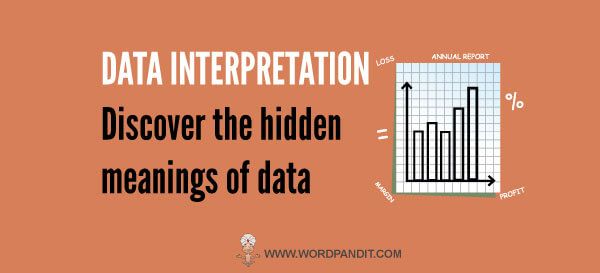## Data Interpretation (Level-2): Set-40

Directions for the Question: Study the following pie-charts to answer these questions. Question 1: If 22% of the Production department persons are posted at Hyderabad region, then what % of Hyderabad workforce are in Production department? (a) 38.5% (b) 78% (c) 68 %...## Data Interpretation (Level-2): Set-39

The pie charts below show the break-up of the total revenue and total sales volume for a company, for the year ended 2003. (Total revenue= Rs. 11,39,200 million and total sales volume= 653610 unit. Based on the data provided in both the figures, answer the question...## Data Interpretation (Level-2): Set-38

Directions 1 to 4 : The table below represents the information collected by TRAI about the Service Area wise Access :f (Wireless + Wire line) subscribers in India. On the basis of the information provided in the table answer the questions nat follow. Question 1. Which...## Data Interpretation (Level-2): Set-37

Directions for questions 1 to 4 : Study the following graph and answer the question that follow Question 1.  If the world energy council formulates a norm for high emission countries to reduce their emission each year by 12.5% for the next two years then what would be...## Data Interpretation (Level-2): Set-36

Directions for questions 15-19: Study the following table carefully and answer the questions Note: Whatever is produced in a year is either used or lost in the production in the same year. Question 1. During which year the Oil used for House Hold as a percentage of...## Data Interpretation (Level-2): Set-35

Direction for question 1– 6: Answer the questions based on the following graphs Question 1.  Which of the following year exhibited highest percentage decrease over the preceding year in the automobile production? A. 2005 B.2006 C.2007 D.2008 Question 2.  Assuming...